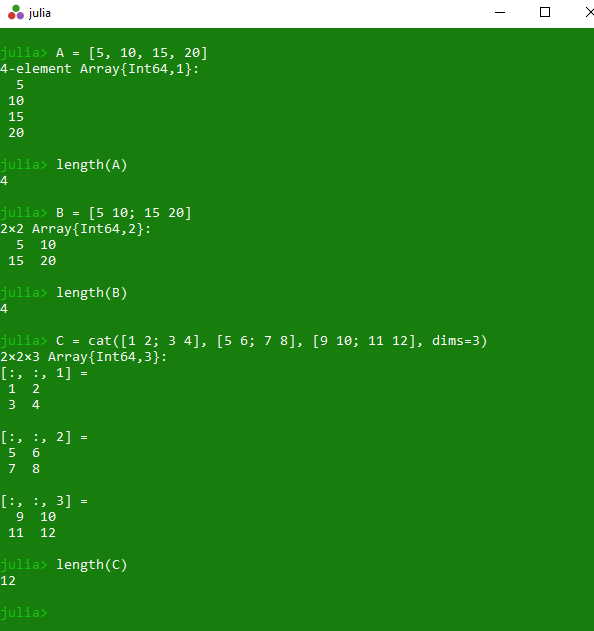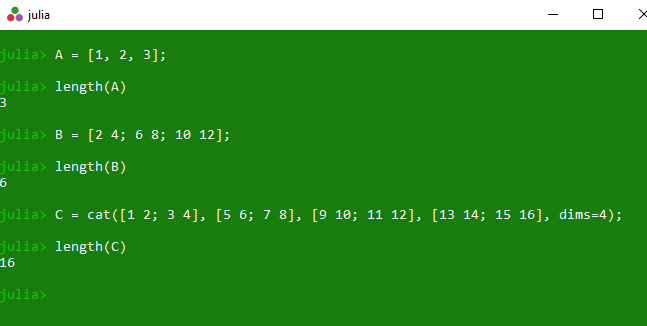# Get number of elements of array in Julia – length() Method

The `length()` is an inbuilt function in julia which is used to return the number of elements present in the specified array.

Syntax: length(A::AbstractArray)

Parameters:

• A: Specified array

Returns: It returns the number of elements present in the specified array.

Example 1:

 `# Julia program to illustrate  ` `# the use of Array length() method ` `  `  `# Finding the number of elements present in ` `# the specified 1D array A. ` `A ``=` `[``5``, ``10``, ``15``, ``20``] ` `println(length(A)) ` `  `  `# Finding the number of elements present in ` `# the specified 2D array B of size 2 * 2 ` `B ``=` `[``5` `10``; ``15` `20``] ` `println(length(B)) ` `  `  `# Finding the number of elements present in ` `# the specified 3D array C of size 2 * 2*2 ` `C ``=` `cat([``1` `2``; ``3` `4``], [``5` `6``; ``7` `8``], ` `        ``[``9` `10``; ``11` `12``], dims ``=` `3``) ` `println(length(C)) `

Output:Example 2:

 `# Julia program to illustrate  ` `# the use of Array length() method ` `   `  `# Finding the number of elements present in ` `# the specified 1D array A. ` `A ``=` `[``1``, ``2``, ``3``]; ` `println(length(A)) ` `   `  `# Finding the number of elements present in ` `# the specified 2D array B of size 2 * 2 ` `B ``=` `[``2` `4``; ``6` `8``; ``10` `12``]; ` `println(length(B)) ` `   `  `# Finding the number of elements present in ` `# the specified 3D array C of size 2 * 2*2 ` `C ``=` `cat([``1` `2``; ``3` `4``], [``5` `6``; ``7` `8``],  ` `        ``[``9` `10``; ``11` `12``], [``13` `14``; ``15` `16``], dims ``=` `4``); ` `println(length(C)) `

Output:Whether you're preparing for your first job interview or aiming to upskill in this ever-evolving tech landscape, GeeksforGeeks Courses are your key to success. We provide top-quality content at affordable prices, all geared towards accelerating your growth in a time-bound manner. Join the millions we've already empowered, and we're here to do the same for you. Don't miss out - check it out now!

Previous
Next